0 and B is the set of all real numbers less than 2. What is A ∩ B?" /> [Placement] CU-AAT Math

# [Placement] CU-AAT Math

First Name:
Last Name:
Email:
Phone:
School:
CU-AAT Math Placement Test 2017 (25 minutes)

*The use of a calculator is NOT permitted*

Press "Next" to start.
CU-AAT Math Placement Test 2017 (25 minutes)

*The use of a calculator is NOT permitted*

Press "Next" to start.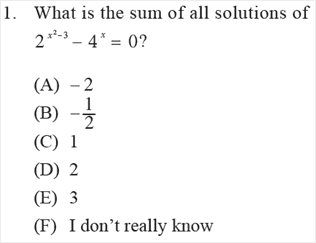(A)
(B)
(C)
(D)
(E)
(F)
2. If A is the set of all solutions of x2 - 1 > 0 and B is the set of all real numbers less than 2. What is A ∩ B?
(A) (-∞,-1] ∪ [1,2)
(B) (-∞,-1) ∪ [1,2)
(C) (-∞,-1) ∪ (1,2)
(D) (-∞,-1] ∪ (1,2)
(E) (-∞,-1] ∪ (1,2]
(F) I don’t really know
3. What is the product of all solutions of (x - 4) (x + 1) = 6?
(A) -15
(B) -10
(C) 6
(D) 10
(E) 15
(F) I don’t really know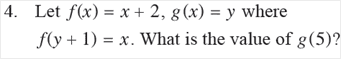(A) 0
(B) 2
(C) 3
(D) 4
(E) 5
(F) I don’t really know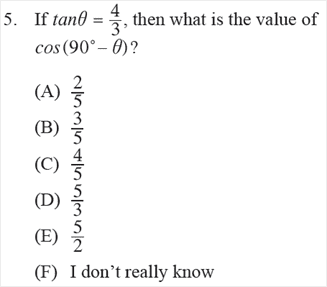(A)
(B)
(C)
(D)
(E)
(F)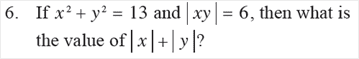(A) 2
(B) 3
(C) 5
(D) 7
(E) 8
(F) I don’t really know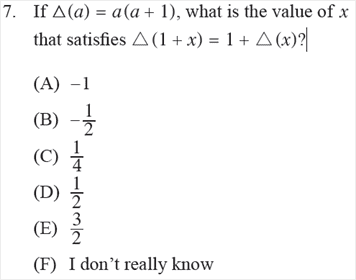(A)
(B)
(C)
(D)
(E)
(F)
8. If A, B, C are the first 3 terms of an arithmetic sequence such that

A + B + C = 54
C - A = 10

What is the fourth term of the sequence?
(A) 23
(B) 26
(C) 28
(D) 29
(E) 31
(F) I don’t really know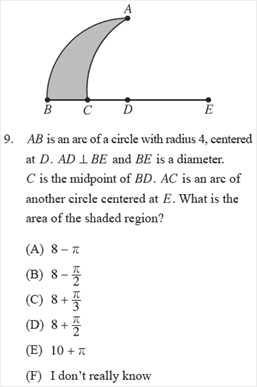(A)
(B)
(C)
(D)
(E)
(F)
10. What is the area of the triangle with vertices (- 5,0), (-3,-4) and (3,4)?
(A) 10
(B) 15
(C) 20
(D) 25
(E) 30
(F) I don’t really know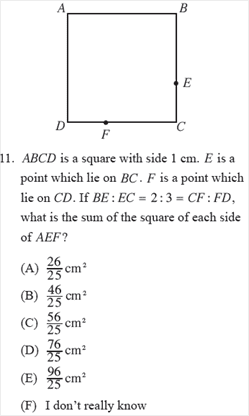(A)
(B)
(C)
(D)
(E)
(F)
12. Which graph of the following relations intercepts the x-axis twice only?
(A) y = x2 + 10x + 25
(B) y = x3 - 3x2 + 2x
(C) y = x4 - x2 - 2
(D) y = x5 + x3
(E) y = x6 + 2x4 + 3
(F) I don’t really know
13. Let an be a sequence such that
a1 + a2 + ... + an = Sn = n3 + n2 + 1
for all positive integers n. What is the difference between a10 and a2?
(A) 250
(B) 275
(C) 280
(D) 295
(E) 305
(F) I don’t really know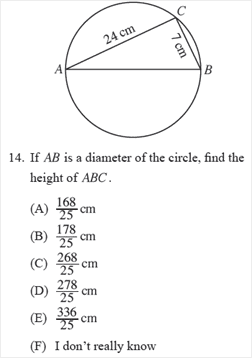(A)
(B)
(C)
(D)
(E)
(F)
15. What is the probability of randomly picking one number from 1, 2, ..., 50 and get a number that is divisible by 3 or 5?
(A) 0.23
(B) 0.46
(C) 0.5
(D) 0.68
(E) 0.82
(F) I don’t really know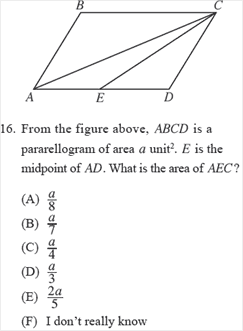(A)
(B)
(C)
(D)
(E)
(F)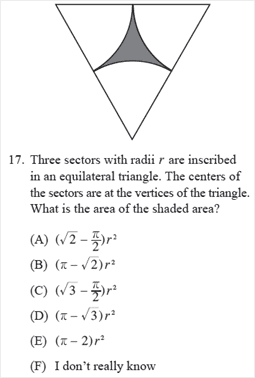(A)
(B)
(C)
(D)
(E)
(F)
18. Let a1 = 1, a2 = 2 , an+1 = 2an-1 - an
where n ≥ 2. What is the average of a5, a6, a7, a8?
(A) 6
(B) 7
(C) 8
(D) 9
(E) 10
(F) I don’t really know(A)
(B)
(C)
(D)
(E)
(F)
20. It takes 6 men 10 days to pack 20 boxes. How many men are needed to pack 30 boxes in 9 days?
(A) 6
(B) 7
(C) 8
(D) 9
(E) 10
(F) I don’t really know
{"name":"[Placement] CU-AAT Math", "url":"https://www.quiz-maker.com/QBSU9L8","txt":"CU-AAT Math Placement Test 2017 (25 minutes) *The use of a calculator is NOT permitted*   Press \"Next\" to start., 2. If A is the set of all solutions of x2 - 1 > 0 and B is the set of all real numbers less than 2. What is A ∩ B?","img":"https://www.quiz-maker.com/3012/images/ogquiz.png"}
Tools: Quiz Maker | Survey Maker